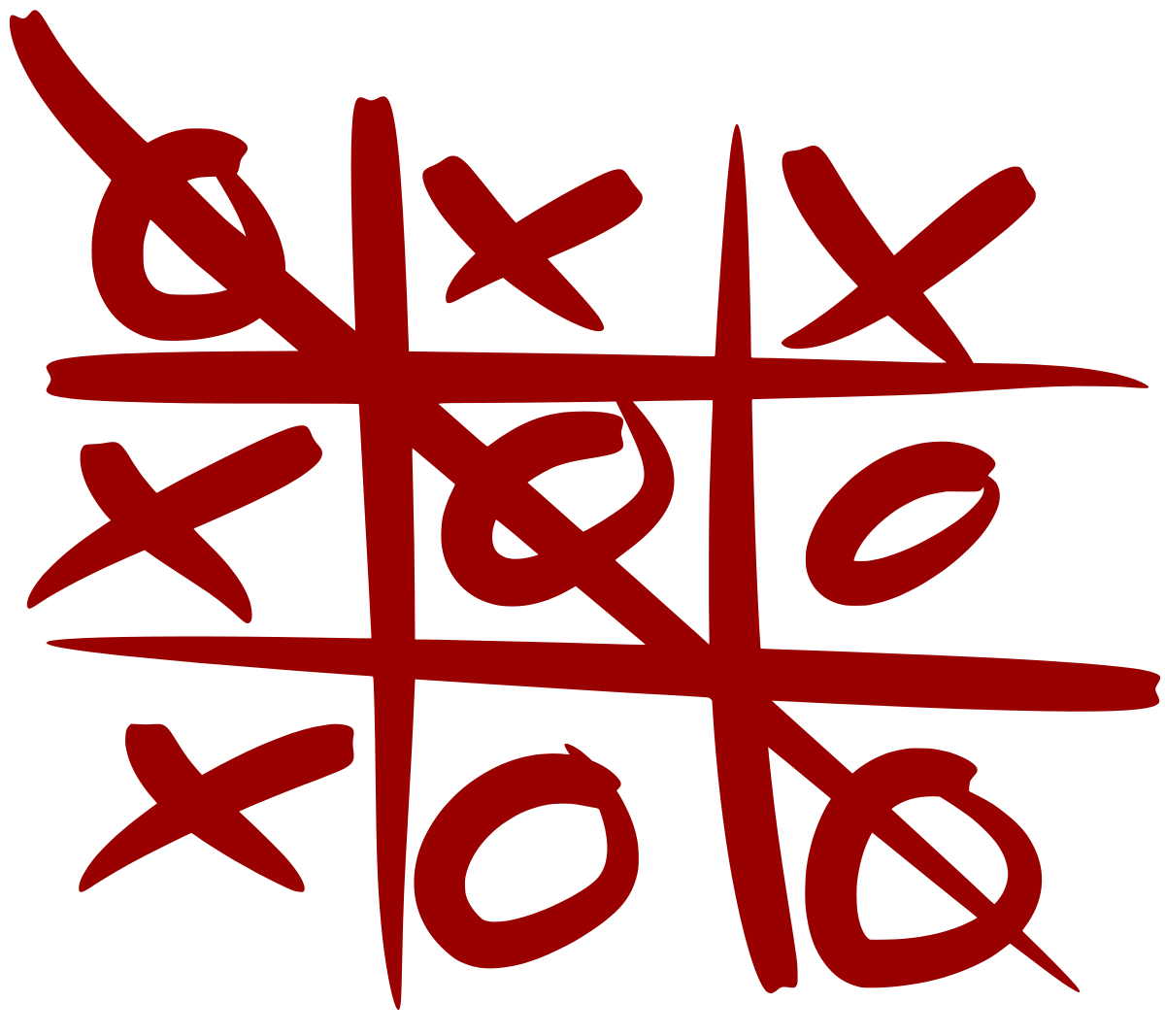#### Problem Statement:

Assume the following rules are for the tic-tac-toe game on an n x n board between two players:

A move is guaranteed to be valid and is placed on an empty block. Once a winning condition is reached, no more moves are allowed. A player who succeeds in placing n of their marks in a horizontal, vertical, or diagonal row wins the game.

Implement the TicTacToe class:
TicTacToe(int n): Initializes the object the size of the board n.
int move(int row, int col, int player): Indicates that player with id player plays at the cell (row, col) of the board. The move is guaranteed to be a valid move.

Example:

Assume that player 1 is "X" and player 2 is "O" in the board.
TicTacToe ticTacToe = new TicTacToe(3);

```ticTacToe.move(0, 0, 1); // return 0 (no one wins)
|X| | |
| | | |    // Player 1 makes a move at (0, 0).
| | | |

ticTacToe.move(0, 2, 2); // return 0 (no one wins)
|X| |O|
| | | |    // Player 2 makes a move at (0, 2).
| | | |

ticTacToe.move(2, 2, 1); // return 0 (no one wins)
|X| |O|
| | | |    // Player 1 makes a move at (2, 2).
| | |X|

ticTacToe.move(1, 1, 2); // return 0 (no one wins)
|X| |O|
| |O| |    // Player 2 makes a move at (1, 1).
| | |X|

ticTacToe.move(2, 0, 1); // return 0 (no one wins)
|X| |O|
| |O| |    // Player 1 makes a move at (2, 0).
|X| |X|

ticTacToe.move(1, 0, 2); // return 0 (no one wins)
|X| |O|
|O|O| |    // Player 2 makes a move at (1, 0).
|X| |X|

ticTacToe.move(2, 1, 1); // return 1 (player 1 wins)
|X| |O|
|O|O| |    // Player 1 makes a move at (2, 1).
|X|X|X|
```

#### Java and Python Solution:

This is a very simple yet interesting problem. While solutioning for this problem we will focus on how we go on optimizing our solution from O(n2) to all the way to O(1).

#### Instructor: